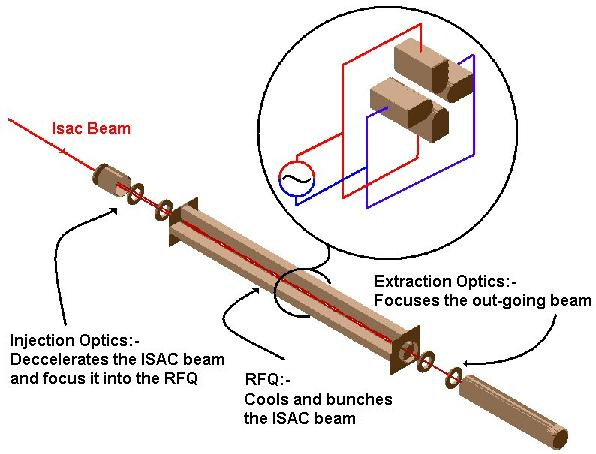Trapping in 2-D – The Radio Frequency Quadrupole Interactive Demonstration of Simulations The Radio Frequency Quadrupole (RFQ) uses time dependent electric fields with alternating polarity to trap ions in two dimensions. These devices are generally used for mass spectrometry and analytical chemistry although in recent years they have become popular tools at online facilities.

A gas filled segmented RFQ device allows one to cool and bunch a beam of ions. Such beams can be manipulated with high efficiency and allow for great accuracy when used in online experiments.

Consider the four electrode structure shown. We place a positive potential on electrodes one and three and a negative potential on electrodes two and four.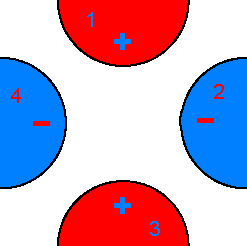Now consider a positive ion placed inside this electrode structure. The ion will feel a repulsive force due to the positive electrodes and an attractive force due to the negative electrodes. The ion will hence be accelerated toward one of the negative electrodes.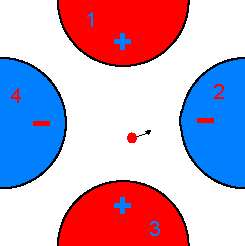However if we switch the potentials on the electrodes, before the ion reaches the negative electrode, such that one and three become negative and two and four become positive the ion will not reach the now positive electrode but will instead be attracted towards one of the new negative potentials. So by switching the potentials we can now trap the ions in 2-D.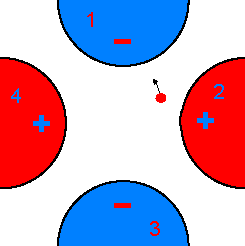Some Math

We require the force on the ion to be proportional to the distance from the center of the trap

 F - r = f(x,y)
 f = f(x2,y2)

So we require a potential proportional to the square of the distance from the center of the trap. The ideal 2-D parabolic potential has the form:

 f = f0 2r02 (x2 - y2)

Such a potential generates a quadrupolar field.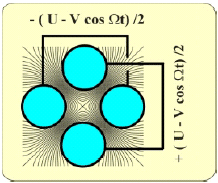So we require a potential proportional to the square of the distance from the center of the trap. The ideal 2-D parabolic potential has the form:

 f = f0 2r02 (x2 - y2)

Such a potential generates a quadrupolar field.A close approximation to this potential is realized by the four electrode geometry shown. Where r = 1.15r0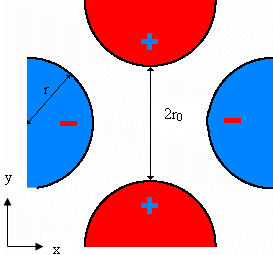Now we require potential to vary as a function of time so we let:

 f0 = Vcos(wt)

So:

 f = V cos ( wt ) 2r02 (x2 - y2)

Using Newton’s second law we obtain the following equations of motion for the ions:

 x + e mr02 ( V cos wt ) x = 0
 y - e mr02 ( V cos wt ) y = 0
 z = 0

We can now extend our trap into 3-D such that it has an extent along the Z-axis. The ion motion along this axis will be unaffected. The ions motion with respect to a radial axis will be a combination of a simple harmonic macro-motion coupled with a micro-motion due to the RF.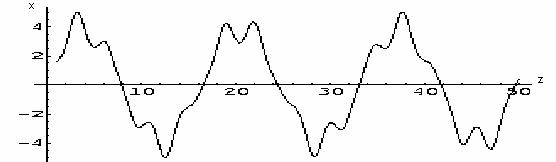The TITAN project will use a segmented gas filled RFQ device in order to cool and bunch the incoming ISAC beam.

Cooling of an Ion Beam in an RFQ

Consider an ion beam that is injected into a box filled with an inert buffer gas. The beam will lose energy via collisions with the gas until, some time later, it comes into equilibrium with the gas. However due to the complete randomness of the cooling process the beam will disperse such that our end product is a box filled with a mixture of two elements.

Consider now the same process in an RFQ. The buffer gas has no charge and hence doesn’t feel any forces due to the RFQ. The ion beam is charged and feels a force that tends to push it onto the RFQ axis. Hence in an RFQ we can cool the beam without dispersion. Since the buffer gas is inert, charge exchange doesn’t take place, hence the ions remain charged and the gas remains neutral.

The bottom diagram shows the motion of an ion in a gas filled RFQ. We can clearly see damping of the radial and longitudinal motions.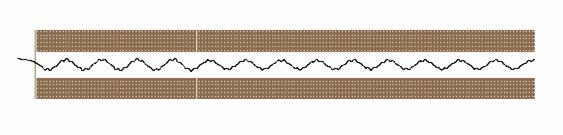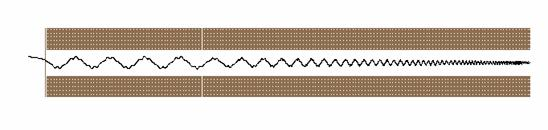The Segmented RFQ

We have seen how a simple RFQ device can be used to trap ions in 2-D. By segmenting such an RFQ device a longitudinal potential can be applied and hence trapping in 3-D achieved. Consider the simple case where each of the rods of the RFQ is segmented into three pieces.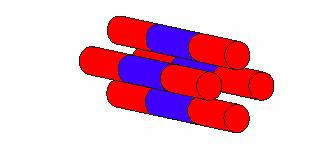By applying a higher potential to the red segments than to the blue segments we create a simple potential well along the length of the trap. We can capture ions in the well which can then be pulsed out by switching the potential on the end rods from high to low voltage.

TITANs RFQ Cooler and Buncher

The TITAN project will use a 70cm RFQ device, ro=10mm, Vpp=10kV, segmented into 24 pieces each separated by 0.5mm. The device will be used to cool and bunch a 60KeV ion beam taken from TRIUMFs ISAC facility.